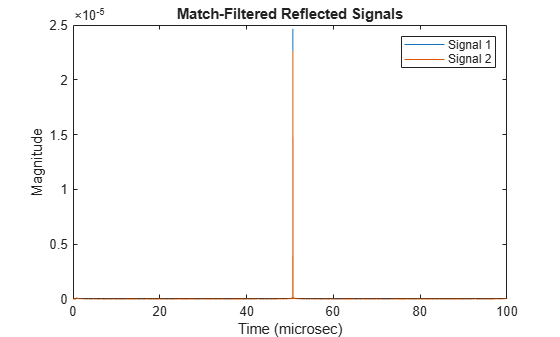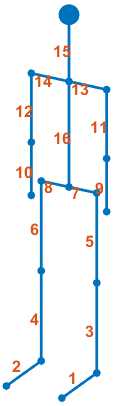# reflect

Reflected signal from walking pedestrian

Since R2019a

## Syntax

``Y = reflect(pedestrian,X,ANG)``

## Description

example

````Y = reflect(pedestrian,X,ANG)` returns the reflected signal, `Y`, from incident signals, `X`, on a pedestrian. The reflected signal is the sum of signals from all body segments. `ANG` defines the directions of the incident and reflected signals with respect to the body segments.```

## Examples

collapse all

Compute the reflected radar signal from a pedestrian moving along the x-axis away from the origin. The radar operates at 24 GHz and is located at the origin. The pedestrian is initially 100 meters from the radar. Transmit a linear FM waveform having a 300 MHz bandwidth. The reflected signal is captured at the moment the pedestrian starts to move and at two seconds into the motion.

Create a linear FM waveform and a free space channel to propagate the waveform.

```c = physconst('Lightspeed'); bw = 300.0e6; fs = bw; fc = 24.0e9; wav = phased.LinearFMWaveform('SampleRate',fs,'SweepBandwidth',bw); x = wav(); channel = phased.FreeSpace('OperatingFrequency',fc,'SampleRate',fs, ... 'TwoWayPropagation',true);```

Create the pedestrian object. Set the initial position of the pedestrian to 100 m on the x-axis with initial heading along the positive x-direction. The pedestrian height is 1.8 m and the pedestrian is walking at 0.5 meters per second.

```pedest = backscatterPedestrian( 'Height',1.8, ... 'OperatingFrequency',fc,'InitialPosition',[100;0;0], ... 'InitialHeading',0,'WalkingSpeed',0.5);```

The first call to the `move` function returns the initial position, initial velocity, and initial orientation of all body segments and then advances the pedestrian motion two seconds ahead.

`[bppos,bpvel,bpax] = move(pedest,2,0);`

Transmit the first pulse to the pedestrian. Create 16 replicas of the signal and propagate them to the positions of the pedestrian body segments. Use the `rangeangle` function to compute the arrival angle of each replica at the corresponding body segment. Then use the `reflect` function to return the coherent sum of all the reflected signals from the body segments at the pedestrian initial position.

```radarpos = [0;0;0]; xp = channel(repmat(x,1,16),radarpos,bppos,[0;0;0],bpvel); [~,ang] = rangeangle(radarpos,bppos,bpax); y0 = reflect(pedest,xp,ang);```

Obtain the position, velocity, and orientation of each body segment then advance the pedestrian motion another two seconds.

`[bppos,bpvel,bpax] = move(pedest,2,0);`

Transmit and propagate the second pulse to the new position of the pedestrian.

```radarpos = [0;0;0]; xp = channel(repmat(x,1,16),radarpos,bppos,[0;0;0],bpvel); [~,ang] = rangeangle(radarpos,bppos,bpax); y1 = reflect(pedest,xp,ang);```

Match-filter and plot both of the reflected pulses. The plot shows the increased delay of the matched filter output as the pedestrian walks away.

```filter = phased.MatchedFilter('Coefficients',getMatchedFilter(wav)); ymf = filter([y0 y1]); t = (0:size(ymf,1)-1)/fs; plot(t*1e6,abs(ymf)) xlabel('Time (microsec)') ylabel('Magnitude') title('Match-Filtered Reflected Signals') legend('Signal 1','Signal 2')```Zoom in and show the time delays for each signal.

```plot(t*1e6,abs(ymf)) xlabel('Time (microsec)') ylabel('Magnitude') title('Matched-Filtered Reflected Signals') axis([50.65 50.7 0 .0026]) legend('Signal 1','Signal 2')```## Input Arguments

collapse all

Pedestrian target model, specified as a `backscatterPedestrian` object.

Incident radar signals on each body segment, specified as a complex-valued M-by-16 matrix. M is the number of samples in the signal. See Body Segment Indices for the column representing the incident signal at each body segment.

Data Types: `double`
Complex Number Support: Yes

Directions of incident signals on the body segments, specified as a real-valued 2-by-16 matrix. Each column of `ANG` specifies the incident direction of the signal to the corresponding body part. Each column takes the form of an azimuth-elevation pair, `[AzimuthAngle;ElevationAngle]`. Units are in degrees. See Body Segment Indices for the column representing the incident direction at each body segment.

Data Types: `double`

## Output Arguments

collapse all

Combined reflected radar signals, returned as a complex-valued M-by-1 column vector. M equals the same number of samples as in the input signal, `X`.

Data Types: `double`
Complex Number Support: Yes

collapse all

### Body Segment Indices

Body segment indices define which columns in `X` and `ANG` contain the data for a specific body segment. For example, column 3 of `X` contains sample data for the left lower leg. Column 3 of `ANG` contains the arrival angle of the signal at the left lower leg.

Body SegmentIndex
Left foot1Right foot2
Left lower leg3
Right lower leg4
Left upper leg5
Right upper leg6
Left hip7
Right hip8
Left lower arm9
Right lower arm10
Left upper arm11
Right upper arm12
Left shoulder13
Right shoulder14